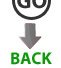# How to calculate precipitation rate

There are two methods to calculate the rate at which sprinklers apply water, or the precipitation rate. The first uses empirical field measurement. The second makes use of published data from sprinkler performance charts

The Measuring Can Method

This is as simple as measuring rainfall. Take a rain gauge, or non tapered cup or can, set it within the area to be watered by the zone being tested. Run the system for 5, 10, or 15 minutes. Measure the amount of water gathered, the rain gauge will have a reading that will indicate the amount of rainfall gathered, the cup or can be measured with a ruler. Usually, rain gauges measure in tenths of inches, rulers measure eighths, quarters, halves, and otherwise.Let’s say you have gathered a tenth of an inch in 10 minutes of watering, therefore, in order to apply one inch of water, you would have to apply water in that area for a total of 100 minutes. To apply one and a half inches of water, which is 15 tenths of water, you would have to apply water in that area for a total of 150 minutes. Measure each zone, try different areas within the zone to be sure water is being evenly distributed. You can run the zone for 5 minutes to speed up the process, but longer run times can give a more accurate result.

Using the Precipitation Rate Formula

In this example, we are using what is called the Total Area Method. This calculation is best suited for determining the average precipitation rate for a system, or a portion of a system, that uses sprinklers with differing arcs, flow rates, and spacings.

The formula for the Total Area Method is:

Pr = 96.25 x Total GPM
Total Area

where: Pr is precipitation rate in inches per hour.

96.25 is a constant that converts gallons per minute to inches
per hour. It is derived from 60 min/hr divided by 7.48
gallons per cu.ft. times 12 inches per foot.

Total GPM is the cumulative flow from all sprinklers in the specified area, in gallons per minute. (from sprinkler charts)

Total Area is the area irrigated, in square feet.

(Note: This information is from the Precipitation Rates and Sprinkler Irrigation - Student Manual, (3/6/96) produced by Hunter Industries, San Marcos, CA.)

Example:

An area, with a total footage of 1000 square feet, is being watered using a total of 10 gallons per minute. Using this method, we can determine the precipitation rate as follows:

Pr = 96.25 x Total GPM
Total Area

= 96.25 x 10
1000

= .96 in./hr.

Related Topics: